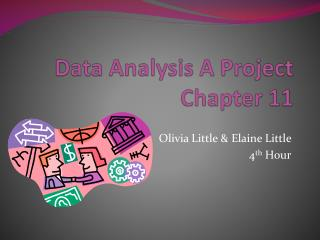DownloadDownload PresentationData Analysis A Project Chapter 11

# Data Analysis A Project Chapter 11

Télécharger la présentation## Data Analysis A Project Chapter 11

- - - - - - - - - - - - - - - - - - - - - - - - - - - E N D - - - - - - - - - - - - - - - - - - - - - - - - - - -
##### Presentation Transcript

1. Data Analysis A Project Chapter 11 Olivia Little & Elaine Little 4th Hour

2. Part 1 Introduction • Our credit limit is \$12,000. • Our previous months balance is \$4,208. • We have to calculate the finance charge for last months balance using unpaid balance method. • We also have to buy and return 5 different items and calculate the finance charge.

3. Credit Limit: \$12,000 • Previous Months Balance: \$4,208 Scottish Fold Kitten (with sales tax): \$250 * 1.06 = \$265 Wii (with sales tax): \$129 * 1.06 = \$136.74 Couch (with sales tax): \$142 * 1.06= \$150.52 Purse (with sales tax): \$39.99 * 1.06= \$42.39 Potted Plant (with sales tax): \$54 * 1.06 = \$57.24 Returned Item

4. Credit Limit: \$12,000Previous Months Balance: \$4,208 Find P 4208 -126.24 (1 Payment) +77.15 (Interest) +265 (Kitten) +136.74 (Wii) +150.52 (Couch) +42.39 (Purse) -57.24 (Plant) =\$4,696.86 I=Prt I=4208*.22*1/12 I=\$77.15 I=Prt I=4696.86 * .22 *1/12 I= \$86.11 New Finance Charge

5. Conclusion of Part 1 • We first multiplied our previous months balance by 22% and 1/12 to get the interest. • We then found the new P by adding interest, purchases and subtracting returns and a payment from the previous months balance. • Lastly we found the new I using the new P.

6. Wayne State University • Tuition is \$10,876.35 • Room and Board is \$9,269 • The total over all Tuition is (10,876.35 * 4) = \$43,505.40 • The total over all Room and Board is (9,269 * 4) = \$37,076 • The total over all is (43,505.40 + 37,076) = \$80,581.40

7. Finding the monthly payment for 4 years at Wayne State Universityusing the future value of an annuity A= R (1 + r/n) ^ nt – 1 / (r/n) 80581.40= R (1+.085/12) ^ 12* 18 -1 / (.085/12) 80581.40= R (136.8214549) R= 588.9529538

8. Finding Monthly Payment at Wayne State: Conclusion • We used the Future Value of an Annuity to solve for R • We plugged in all the information and then calculated the information and divided both sides by the calculation. • The monthly payments for 18 years would come out to be about \$588.95 • These findings are extremely high but compared to other schools it’s not as much, especially since it’s including room and board.

9. Chrysler 200 \$17,988.20

10. Price with tax: \$16,970 * 1.06 = \$17,988.20 Interest Charged on Car: I=Prt I= 17988.20 * .09 * 5 I= \$8,094.69 Monthly Payments: MP=(P + I)/n MP=(17988.20 + 8094.69) / 5*12 MP=\$434.71

11. Car Conclusion • First we used the price of the car and multiplied it by 1.06 to add tax. • Then we found the interest charged on the car by using add on interest method. • After finding interest, we found the monthly payments.

12. THE END